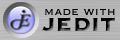# Polynomial Roots

Enter the constants of your polynomial here:

• X5
• X4
• X3
• X2
• X
Use Hessenberg:

Math::Polynomial::Solve is a module that solves polynomial equations by either numerical formulae or by iterative methods.

Polynomials of degrees two, three, and four will be solved by numerical methods unless you click on the "Use Hessenberg" check boxn. Solving for a quintic equation will force the use of the QR Hessenberg method even if you don't click on the check box.One of my sources was R. W. D. Nickalls's paper, "A New Approach to solving the cubic: Cardan's solution revealed," The Mathematical Gazette, 77, 354-359, 1993. Dr. Nickalls was kind enough to send me an updated version of his article, which is available in PDF format by clicking here.